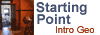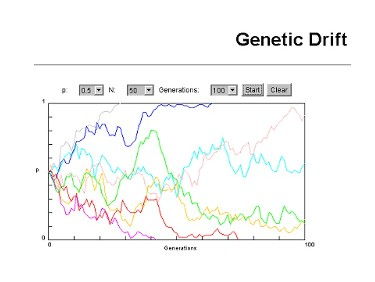# Genetic DriftThis material was originally created for Starting Point:Introductory Geology
and is replicated here as part of the SERC Pedagogic Service.

#### Summary

In this biology simulation, students use a mathematical simulation of genetic drift to answer questions about the factors that influence this evolutionary process. Students run a series of simulations varying allele frequency and population size and then analyze their data and propose a model to explain their results. A second set of simulations is performed with natural selection added to the simulation.Used this activity? Share your experiences and modifications

## Learning Goals

• Students analyze data and use this data to form a model of how genetic drift works.
• Students explain how population size and allele frequency and could contribute to loss of an allele in a population.

## Context for Use

This could be used in lecture as a demonstration, or an interactive activity where the teacher runs the simulations and the students record the data and answer the questions. It would probably be most effective as an out of class assignment. It could also be integrated into a laboratory.

## Description and Teaching Materials

Students are given a handout with links to two simulations on Genetic Drift. They are then asked to set two parameters (population size and allele frequency) to nine different combinations and run each simulation eight times. The data are then recorded in a table, keeping track of how many simulations had a p=1, 1>p>0, and p=0. P=1 and p=0 indicate the loss of an allele (a or A respectively). This is a crude measure of genetic drift, but it is difficult to pull actual numbers from the graphs.

The students then use a second simulation that has Natural Selection for the A allele incorporated. They run this simulation at three different N values and a fixed allele frequency and compare the results to the first set of simulations.

Finally, there are a collection of multiple choice and short answer questions that ask students to analyze the data and propose a model. Genetic Drift Assignment (Microsoft Word 82kB Jul25 06)

## Teaching Notes and Tips

Students should have had a good description of allele frequencies in class before doing this exercise. Some understanding of population biology might help.

## Assessment

Summative questions are included on the worksheet. They could also be used as formative questions in class or lab if you wanted to give the students the time to work through analyzing the data in class.

## References and Resources

MERLOT description of the Genetic Drift simulation used for the first part of the exercise.

MERLOT description of the Genetic Drift and Natural Selection simulation used for the second part of the exercise.